1594278000

# Confidence Interval, Calculation, and Characteristics

The Confidence Interval (CI) is very important in statistics and data science. In this article, I am going to explain the confidence interval, how to calculate it, and the important characteristics of it.

The confidence interval (CI) is a range of values. It is expressed as a percentage and is expected to contain the best estimate of a statistical parameter. A confidence interval of 95% mean, it is 95% certain that our population parameter lies in between this confidence interval.

## Interpretation of confidence intervals

Here is a statement:

“In a sample of 659 parents with toddlers, 540, about 85 percent, stated they use a car seat for all travel with their toddler. From these results, a 95% confidence interval was provided, going from about 82.3 percent up to 87.7 percent.”

This statement means, it is 95% certain that the population proportion that uses a car seat for all travel with their toddler is 82.3 and 87.7. If we take several subsamples from this population, 95% of the time, the population proportion that uses a car seat for all travel with their toddler will fall between 82.3% to 87.7%.

Can we say that the confidence interval (82.3, 87.7) contains the true population proportion? The answer is unknown. The population proportion is a fixed value but unknown. It is important to remember that 95% confidence does not mean a 95% probability.

## Why Confidence Interval Is Important?

It is important because it is not possible to take data from every single person in a population most of the time. In the example above, the sample size was 659. We estimated the population proportion of the parents with toddlers who use a car seat for all travel from a sample of 659 parents. We could not get the data from all the parents with toddlers. So, we calculate the population proportion from our available sample and consider a margin of error. With that margin of error, we get a range. This range is called a confidence interval. A confidence interval is a way to express how well the sample data represent the total population. You can calculate a confidence interval of any number(less than 100%). But a 95% confidence interval is the most common.

## How to Calculate the Confidence Interval

The formula for the confidence interval is: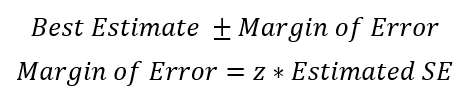We normally want a high confidence level such as 75%, 95%, or 99%. Higher the confidence level(CL), lower the precision. In the example above, the best estimate is 85%. We can calculate the estimates SE from the following formula: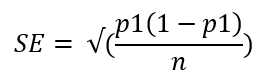In the equation, above p1 is the best estimate and n is the sample size. Here is a table for z- score for a few commonly used confidence level.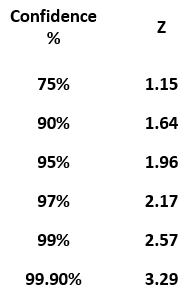Plugging in all the values,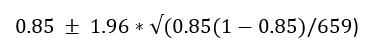The confidence interval come out to be 82.3% and 87.7%.

## The range of CI is higher for higher CL

In the same way, we can calculate a 99% confidence level. You only need to change the z-score. From the table above, the z-score for a 99% confidence level is 2.57. Plugging in that value in the confidence interval formula, the confidence interval for a 99% confidence level is 81.43% to 88.57%. The range of a confidence interval is higher for a higher confidence level.

#statistical-analysis #confidence-interval #statistics #statistical-learning #data-science #data analysis

## Buddha Community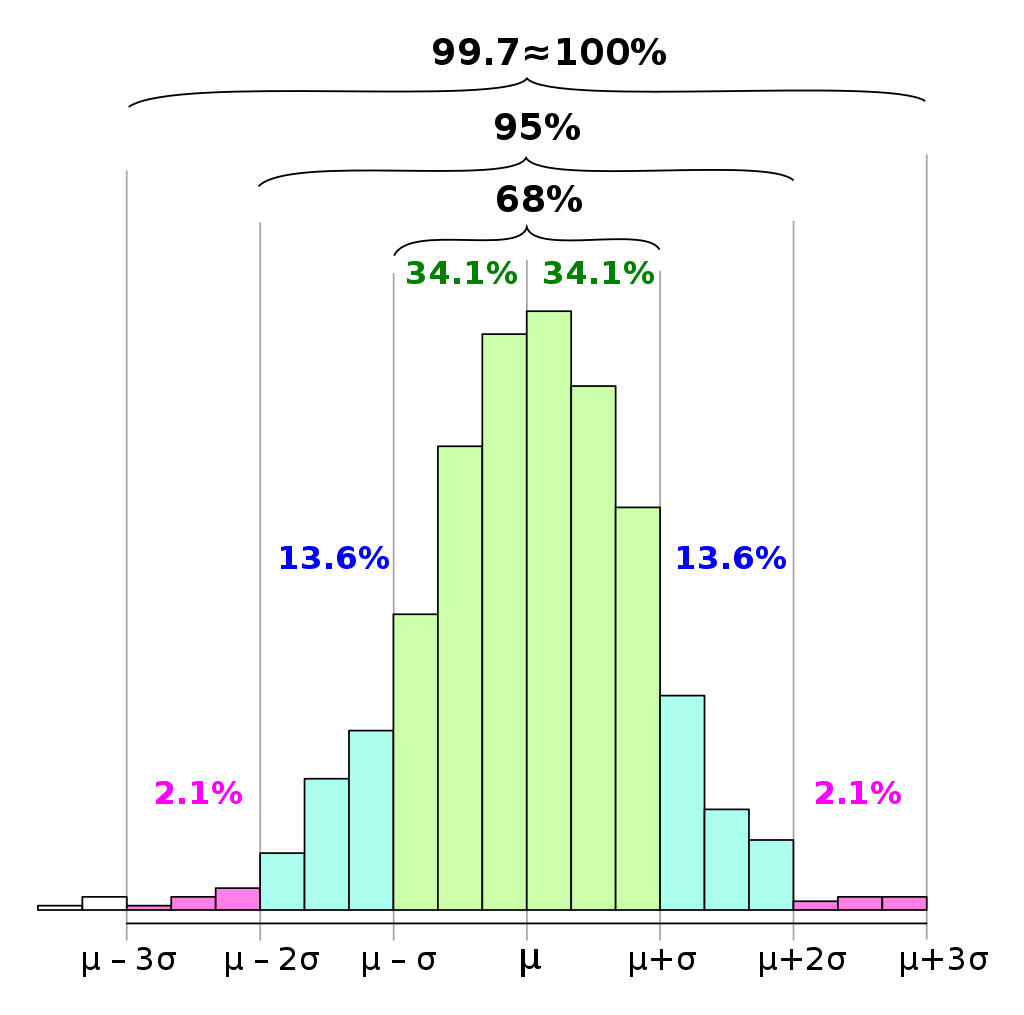1624597948

## Introduction

In a series of weekly articles, I will cover some important statistics topics with a twist.

The goal is to use Python to help us get intuition on complex concepts, empirically test theoretical proofs, or build algorithms from scratch. In this series, you will find articles covering topics such as random variables, sampling distributions, confidence intervals, significance tests, and more.

At the end of each article, you can find exercises to test your knowledge. The solutions will be shared in the article of the following week.

Articles published so far:

As usual, the code is available on my GitHub.

#python #statistics #data-science #machine-learning #confidence intervals with python #confidence intervals1594278000

## Confidence Interval, Calculation, and Characteristics

The Confidence Interval (CI) is very important in statistics and data science. In this article, I am going to explain the confidence interval, how to calculate it, and the important characteristics of it.

The confidence interval (CI) is a range of values. It is expressed as a percentage and is expected to contain the best estimate of a statistical parameter. A confidence interval of 95% mean, it is 95% certain that our population parameter lies in between this confidence interval.

## Interpretation of confidence intervals

Here is a statement:

“In a sample of 659 parents with toddlers, 540, about 85 percent, stated they use a car seat for all travel with their toddler. From these results, a 95% confidence interval was provided, going from about 82.3 percent up to 87.7 percent.”

This statement means, it is 95% certain that the population proportion that uses a car seat for all travel with their toddler is 82.3 and 87.7. If we take several subsamples from this population, 95% of the time, the population proportion that uses a car seat for all travel with their toddler will fall between 82.3% to 87.7%.

Can we say that the confidence interval (82.3, 87.7) contains the true population proportion? The answer is unknown. The population proportion is a fixed value but unknown. It is important to remember that 95% confidence does not mean a 95% probability.

## Why Confidence Interval Is Important?

It is important because it is not possible to take data from every single person in a population most of the time. In the example above, the sample size was 659. We estimated the population proportion of the parents with toddlers who use a car seat for all travel from a sample of 659 parents. We could not get the data from all the parents with toddlers. So, we calculate the population proportion from our available sample and consider a margin of error. With that margin of error, we get a range. This range is called a confidence interval. A confidence interval is a way to express how well the sample data represent the total population. You can calculate a confidence interval of any number(less than 100%). But a 95% confidence interval is the most common.

## How to Calculate the Confidence Interval

The formula for the confidence interval is:We normally want a high confidence level such as 75%, 95%, or 99%. Higher the confidence level(CL), lower the precision. In the example above, the best estimate is 85%. We can calculate the estimates SE from the following formula:In the equation, above p1 is the best estimate and n is the sample size. Here is a table for z- score for a few commonly used confidence level.Plugging in all the values,The confidence interval come out to be 82.3% and 87.7%.

## The range of CI is higher for higher CL

In the same way, we can calculate a 99% confidence level. You only need to change the z-score. From the table above, the z-score for a 99% confidence level is 2.57. Plugging in that value in the confidence interval formula, the confidence interval for a 99% confidence level is 81.43% to 88.57%. The range of a confidence interval is higher for a higher confidence level.

#statistical-analysis #confidence-interval #statistics #statistical-learning #data-science #data analysis1606912089

## How to create a calculator using javascript - Pure JS tutorials |Web Tutorials

### In this video I will tell you How to create a calculator using javascript very easily.

#how to build a simple calculator in javascript #how to create simple calculator using javascript #javascript calculator tutorial #javascript birthday calculator #calculator using javascript and html1592564040

## How to Calculate Confidence Interval of Mean and the Difference of Mean

The confidence interval(CI) of the mean is the process of estimating a range that includes the true mean. For example, a confidence interval of the mean is 3.6. The confidence interval can be 3.2 and 4.1. In statistics and data science, this measure is important. Because it is not possible to take the data from every single sample of the population. So, in most cases, we collect the data from the part of the population. Then estimate the true mean from that part of a population. That’s why a range makes more sense.
I am assuming that you know what confidence interval is and the basics. If not, please check these two articles on Confidence Interval and Calculation of Confidence Interval for Population Proportion.

#statistics #data-analytics #python #data-science #confidence-interval1597824000

## Calculating Confidence Interval with Bootstrapping

### Hi everyone,

In this article, I will attempt to explain how we can find a confidence interval by using Bootstrap Method. Statistics and Python knowledge are needed for better understanding.

Before diving into the method, let’s remember some statistical concepts.

**Variance: **It is obtained by the sum of squared distances between a data point and the mean for each data point divided by the number of data points.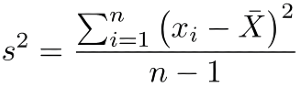Sample variance

Standard Deviation: It is a measurement that shows us how our data points spread out from the mean. It is obtained by taking the square root of the variance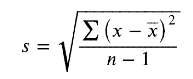Sample standard deviation

Cumulative Distribution Function: It can be used on any kind of variable X(discrete, continuous, etc.). It shows us the probability distribution of a variable. Therefore allowing us to interpret the probability of a value less than or equal to x from a given probability distribution

Empirical Cumulative Distribution Function: Also known as Empirical Distribution Function. The only difference between CDF and ECDF is, while the former shows us the hypothetical distribution of any given population, the latter is based on our observed data.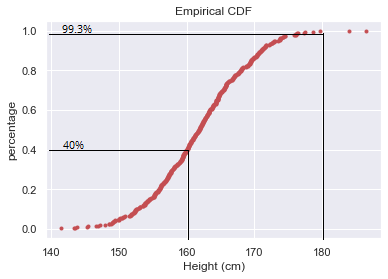For example, how can we interpret the ECDF of the data shown on the chart above? We can say that 40% of heights are less than or equal to 160cm. Likewise, the percentage of people with heights of less than or equal to 180 cm is 99.3%

Probability Density Function: It shows us the distribution of continuous variables. The area under the curve gives us the probability so that the area must always be equal to 1

Normal Distribution: Also known as Gaussian Distribution. It is the most important probability distribution function in statistics which is bell-shaped and symmetric.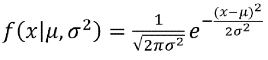Normal (Gaussian) Distribution

**_Confidence Interval: _**It is the range in which the values likely to exist in the population. It is estimated from the original sample and usually defined as 95% confidence but it may differ. You can consider the figure below which indicates a 95% confidence interval. The lower and upper limits of confidence interval defined by the values corresponding to the first and last 2.5th percentiles.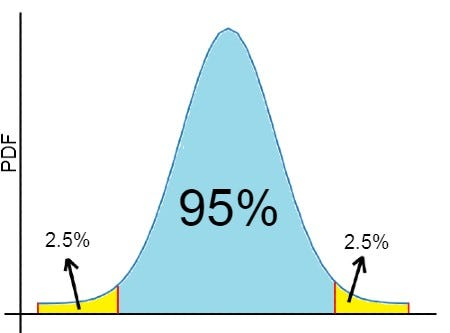95% Confidence Interval, Image by author

## What is Bootstrap Method?

Bootstrap Method is a resampling method that is commonly used in Data Science. It has been introduced by Bradley Efron in 1979. Mainly, it consists of the resampling our original sample with replacement (Bootstrap Sample) and generating Bootstrap replicates by using Summary Statistics.

## Confidence Interval of people heights

In this article, we are going to work with one of the datasets in Kaggle. It is Weight-Height data sets. It contains height (in inches) and weight (in pounds) information of 10.000 people separated by gender.

If you would like to see the whole code, you can find the IPython notebook via this link.

We are going to use only heights of 500 randomly selected people and compute a 95% confidence interval by using Bootstrap Method